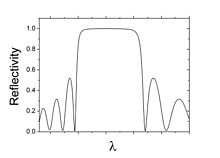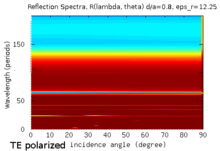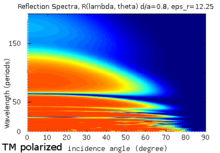# Distributed Bragg reflector

﻿
Distributed Bragg reflector

A distributed Bragg reflector (DBR) is a reflector used in waveguides, such as optical fibers. It is a structure formed from multiple layers of alternating materials with varying refractive index, or by periodic variation of some characteristic (such as height) of a dielectric waveguide, resulting in periodic variation in the effective refractive index in the guide. Each layer boundary causes a partial reflection of an optical wave. For waves whose wavelength is close to four times the optical thickness of the layers, the many reflections combine with constructive interference, and the layers act as a high-quality reflector. The range of wavelengths that are reflected is called the photonic stopband. Within this range of wavelengths, light is "forbidden" to propagate in the structure.

## ReflectivityCalculated reflectivity of a schematic DBR structure

The DBR's reflectivity, R, for intensity is approximately given by$R= \left[\frac{n_o (n_2)^{2N} - n_s (n_1)^{2N}}{n_o (n_2)^{2N} + n_s (n_1)^{2N}}\right]^2,$

where$n_o,\ n_1,\ n_2$ and$n_s\,$ are the respective refractive indices of the originating medium, the two alternating materials, and the terminating medium (i.e. backing or substrate); and N is the number of repeated pairs of low/high refractive index material.

The bandwidth Δλ0 of the photonic stopband can be calculated by$\Delta\lambda_0 = \frac{4\lambda_o}{\pi}\arcsin\left(\frac{n_2 - n_1}{n_2 + n_1}\right),$

where λo is the central wavelength of the band.

Increasing the number of pairs in a DBR increases the mirror reflectivity and increasing the refractive index contrast between the materials in the Bragg pairs increases both the reflectivity and the bandwidth. A common choice of materials for the stack is titanium dioxide (n≈2.5) and silica (n≈1.5). Substituting into the formula above gives a bandwidth of about 200 nm for 630 nm light.

Distributed Bragg reflectors are critical components in vertical cavity surface emitting lasers and other types of narrow-linewidth laser diodes such as distributed feedback lasers. They are also used to form the cavity resonator (or optical cavity) in fiber lasers and free electron lasers.

### TE and TM mode reflectivityCalculated reflectivity for TE mode light at various incidence angles, and wavelengths. Red regions correspond to R=1, while blue regions correspond to R=0, and other colors 0 < R < 1.Calculated reflectivity for TM mode light at various incidence angles, and wavelengths. Orange regions correspond to R=1, while blue regions correspond to R=0, and other colors 0 < R < 1.

This section discusses the interaction of transverse electric (TE) and transverse magnetic (TM) polarized light with the DBR structure, over several wavelengths and incidence angles. This reflectivity of the DBR structure (described below) was calculated using the transfer-matrix method (TMM), where the TE mode alone is highly reflected by this stack, while the TM modes are passed through. This also shows the DBR acting as a polarizer.

For TE and TM incidence we have the reflection spectra of a DBR stack, corresponding to a 6 layer stack of dielectric contrast of 11.5, between an air and dielectric layers. The thicknesses of the air and dielectric layers are 0.8 and 0.2 of the period, respectively. The wavelength in the figures below, corresponds to multiples of the cell period.

This DBR is also a simple example of a 1D photonic crystal. It has a complete TE band gap, but only a pseudo TM band gap.

Wikimedia Foundation. 2010.

### Look at other dictionaries:

• Distributed Bragg reflector — Berechnete Reflektivität eines idealen DBRs Der englischsprachige Begriff distributed Bragg reflector (DBR) bezeichnet einen effizienten Reflektor, der in Lichtleitern oder in optischen Resonatoren eingesetzt wird. Er besteht aus alternierenden,… …   Deutsch Wikipedia

• Distributed Feedback Laser — (englisch, dt. Laser mit verteilter Rückkopplung), im Deutschen meist nur DFB Laser genannt, sind Laserdioden, in denen das aktive Material periodisch strukturiert ist. Die Strukturen wechselnder Brechzahl bilden ein eindimensionales… …   Deutsch Wikipedia

• Bragg diffraction — (also referred to as the Bragg formulation of X ray diffraction) was first proposed by William Lawrence Bragg and William Henry Bragg in 1913 in response to their discovery that crystalline solids produced surprising patterns of reflected X rays… …   Wikipedia

• Bragg's law — In physics, Bragg s law is the result of experiments into the diffraction of X rays or neutrons off crystal surfaces at certain angles, derived by physicist Sir William Lawrence Bragg [ There are some sources, like the Academic American… …   Wikipedia

• Fiber Bragg grating — A fiber Bragg grating (FBG) is a type of distributed Bragg reflector constructed in a short segment of optical fiber that reflects particular wavelengths of light and transmits all others. This is achieved by adding a periodic variation to the… …   Wikipedia

• DBR — Distributed Bragg Reflector (Academic & Science » Electronics) * Destroyed Beyond Repair (Governmental » Military) * Data Base Requirements (Computing » General) * Data Base Requirements (Governmental » Military) * Dumbo Brooklyn Reproductions… …   Abbreviations dictionary

• DBR — Distributed Bragg Reflector Contributor: CASI …   NASA Acronyms

• Volume hologram — Volume holograms are holograms where the thickness of the recording material is much larger than the light wavelength used for recording. In this case diffraction of light from the hologram is possible only as Bragg diffraction, i.e., the light… …   Wikipedia

• Tunable laser — A tunable laser is a laser whose wavelength of operation can be altered in a controlled manner. While all laser gain media allow small shifts in output wavelength, only a few types of lasers allow continuous tuning over a significant wavelength… …   Wikipedia

• Vertical-cavity surface-emitting laser — The vertical cavity surface emitting laser (VCSEL; [v ɪxl] ) is a type of semiconductor laser diode with laser beam emission perpendicular from the top surface, contrary to conventional edge emitting semiconductor lasers (also in plane lasers)… …   Wikipedia